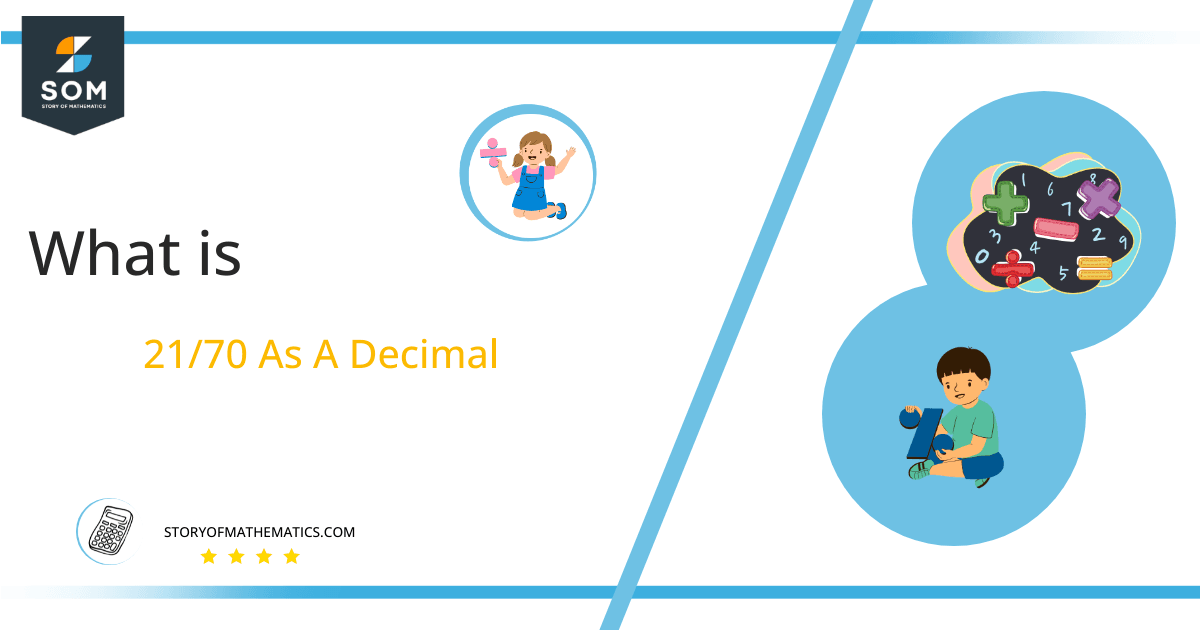# What Is 21/70 as a Decimal + Solution With Free Steps

The fraction 21/70 as a decimal is equal to 0.3.

The Long division method is used to convert a fractional form of division representation into a decimal form. Here, the numerator is the dividend and the denominator is the divisor. The quotient is the result found at the end of the process along with a remainder value that depends on the numbers being divided.

Here, we are more interested in the division types that result in a Decimal value, as this can be expressed as a Fraction. We see fractions as a way of showing two numbers having the operation of Division between them that result in a value that lies between two Integers.Now, we introduce the method used to solve said fraction to decimal conversion, called Long Division, which we will discuss in detail moving forward. So, let’s go through the Solution of fraction 21/70.

## Solution

First, we convert the fraction components, i.e., the numerator and the denominator, and transform them into the division constituents, i.e., the Dividend and the Divisor, respectively.

This can be done as follows:

Dividend = 21

Divisor = 70

Now, we introduce the most important quantity in our division process: the Quotient. The value represents the Solution to our division and can be expressed as having the following relationship with the Division constituents:

Quotient = Dividend $\div$ Divisor = 21 $\div$ 70

This is when we go through the Long Division solution to our problem. Given is the long division process in Figure 1:Figure 1

## 21/70 Long Division Method

We start solving a problem using the Long Division Method by first taking apart the division’s components and comparing them. As we have 21 and 70, we can see how 21 is Smaller than 70, and to solve this division, we require that 21 be Bigger than 70.

This is done by multiplying the dividend by 10 and checking whether it is bigger than the divisor or not. If so, we calculate the Multiple of the divisor closest to the dividend and subtract it from the Dividend. This produces the Remainder, which we then use as the dividend later.

Now, we begin solving for our dividend 21, which after getting multiplied by 10 becomes 210.

We take this 210 and divide it by 70; this can be done as follows:

210 $\div$ 70 $\approx$ 3

Where:

70 x 3 = 210

This will lead to the generation of a Remainder equal to 210 – 210 = 0.

Finally, we have a Quotient generated as 0.3, with a Remainder equal to 0.Images/mathematical drawings are created with GeoGebra.